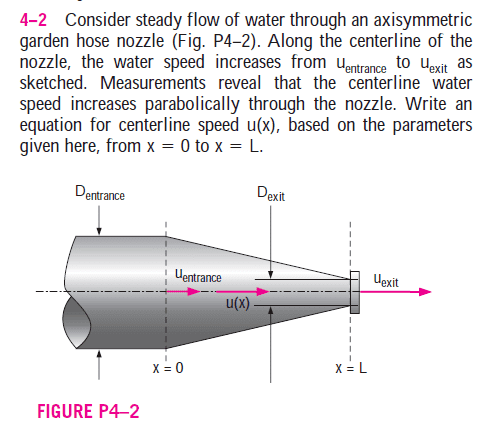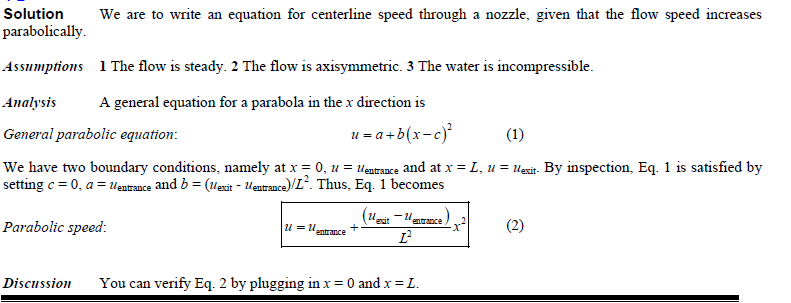# Finding centerline speed of a flow through a nozzle

## Homework Statement## Homework Equations

u=a+ b(x-c)^2

We have two boundary conditions at x=0, u=u(entrance) and x=L, u=u(exit)

Source: Fluid Mechanics by Çengel/Cimbala

## The Attempt at a Solution

I cannot understand the parabolic equation in the x-direction.This is the original solution but it is still hard to understand for me. First I cannot understand general parabolic equation as 1 and why is cet set to 0, a=uentrance and b=(uexit-uentrance)/L^2.

Would you like to explain them?

Thank you.

SteamKing
Staff Emeritus
Homework Helper
View attachment 99692

This is the original solution but it is still hard to understand for me. First I cannot understand general parabolic equation as 1 and why is cet set to 0, a=uentrance and b=(uexit-uentrance)/L^2.

Would you like to explain them?

Thank you.
Did you set x = 0? What speed did you obtain?

Did you set x = L? What speed did you obtain there?

What about some intermediate location, say x = L/2? What is the speed there?

for x=0, u=a+b*(0-c)^2, u=a+b*(c^2)

for x=L, u=a+b*(L-c)^2, u=a+b*(L^2 -2Lc + c^2)

for x=L/2, u=a+b*(L/2 - c)^2, u=a+ b*(L^2 /4 - Lc +c^2)

SteamKing
Staff Emeritus
Homework Helper
for x=0, u=a+b*(0-c)^2, u=a+b*(c^2)

for x=L, u=a+b*(L-c)^2, u=a+b*(L^2 -2Lc + c^2)

for x=L/2, u=a+b*(L/2 - c)^2, u=a+ b*(L^2 /4 - Lc +c^2)
No, you use Eq. (2) and make the substitutions there. Eq. (1) is just a generic parabola.

Meanwhile, would you like to guide me how I can use Latext more effecitively? For example, a link.

Thank you.

Last edited:
SteamKing
Staff Emeritus